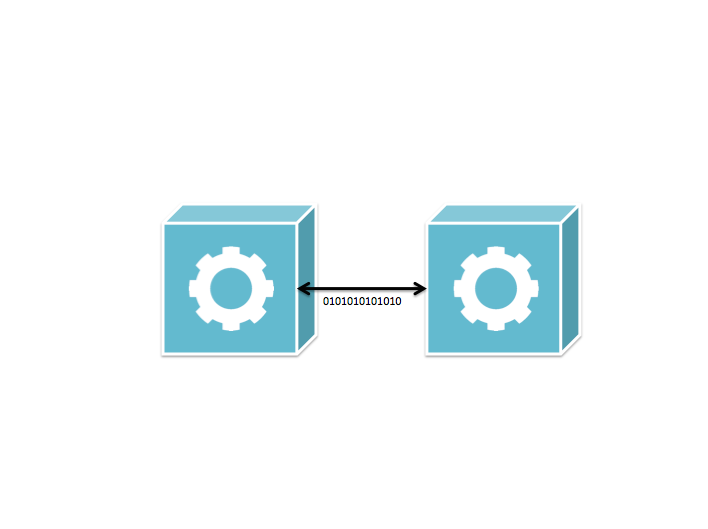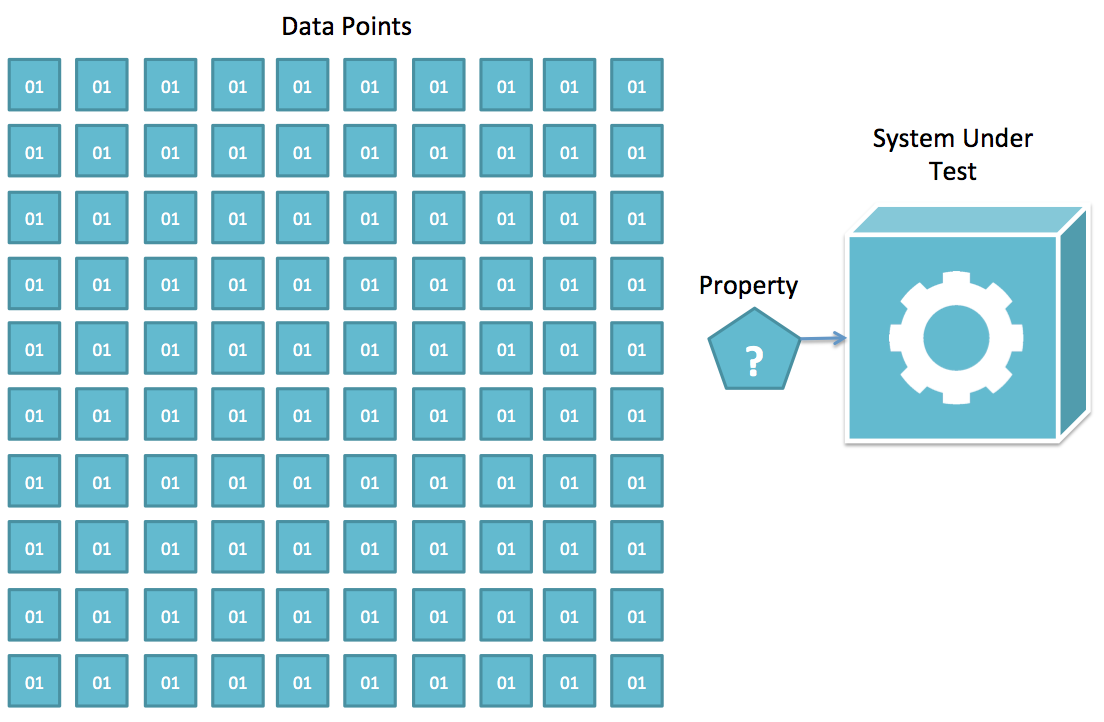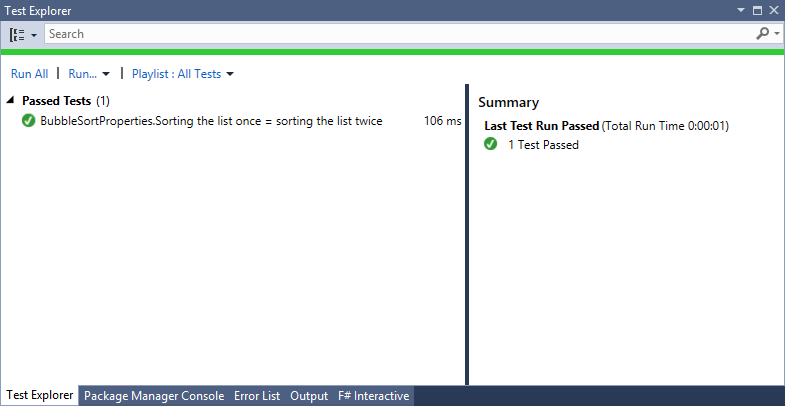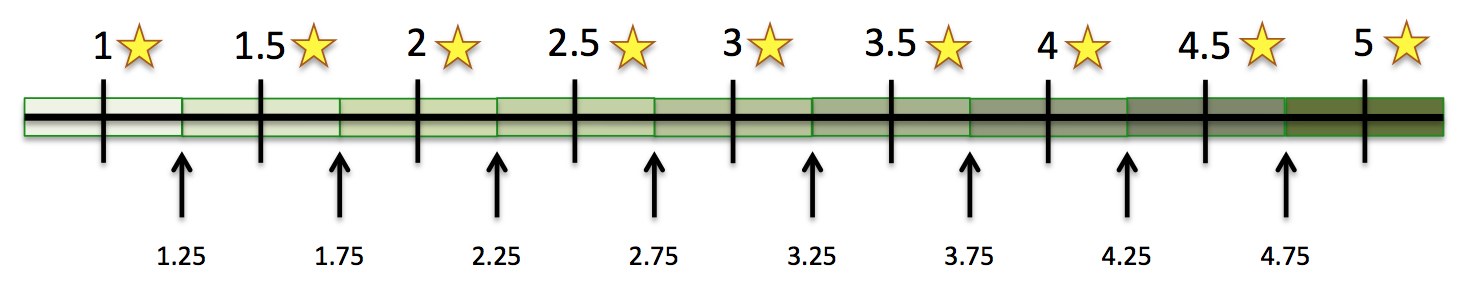# Property-Based Testing with F#

This post is based on a talk I did at Valtech Tech Day, 4 June 2015. You can find the slides for this talk here and the code examples in a zip archive here.

See the writeup for this whole talk in english below the video and slides.

## Introduction

Before diving into property-based testing, let's do a recap of testing in sofware development today.

### Unit Testing

The purpose of unit testing it so verify the correctness of our code. We write tests that verify that the code of our system under test (SUT) is doing what we expect it to. We also write unit tests to drive good design from our code. The unit tests forces design patterns on a macro level like separation of concerns and low coupling / high cohesion.An example of unit testing is where a test verify that our code set status to 'Committed' after committing a transaction.

```public class CommitTransactionShould
{
[Test]
public void SetStatusToCommitted()
{
// AAA
}
}```

Summary

• Unit testing is white box testing
• Unit testing specifies what your code is supposed to do
• Unit testing affects your code design on a macro level

### Integration Testing

The point of integration testing is to verify how our system depends on other systems and how other systems will affect or system.In the follwing integration test scenario we're testing how the database AutoIncremented ID affects our system's entities.

```public class SaveToDatabaseShould
{
[Test]
public void ReturnEntityWithAutoIncrementedID()
{
// AAA
}
}```

Summary

• We use integration tests to verify how our system depends on other systems
• We use integration tests to verify how our system is affected by other systems

### Functional Testing

The functional tests that we write operate on a higher abstraction level. The point of these tests is to specify what's expected of the features, and what functionality features are expected to sustain. This makes functional tests the obvious choice for regression testing, as they are the first thing to break when the feature is no longer fulfilling its promise.One way to do functional testing is by executable specification, of which this is an example of.

```Feature: Order Checkout

Scenario: Pushing order button gives confirmation with order number
Given an order at the order checkout page
And invoice is selected as payment option
When order button is clicked
Then order confirmation should be displayed
And order number should be visible```

Summary

• Functional tests works well as regression tests
• Functional tests works well as documentation
• Functional tests are closer to the user story that is being developed

### Manual Testing

Developers often underestimate manual testing, because they think they're doing it, but in fact they're only checking that their feature is rendered correctly in the browser.

Instead, manual testing is perfomed by a person called 'tester' who is experimenting with the system to find out if it solves the problem it was designed to solve. This doesn't mean that a developer can't be a tester, any person could be a tester, but a developer is not suitable to be a tester for a system he is developing.In contrary to the act of writing tests (unit, integration, functional) the manual testing is a creative task. Automated tests are a specification of what is going to be written, or what has already been written. Manual testing is the act of exploring, investigating and challeging status quo of the system under test.

# Property-Based Testing™

This is where property-based testing comes into play. It is a tool for us developers to think about or system like a manual tester and challenge its functionality. We do this by defining properties for our system.

A property is a high-level specification of behavior that should hold for a range of data points.

In more concrete terms, we state something that is true about our system, and this should be true for any kind of data we feed that statement with.## Example of a property

Let's say that our system is a sort algorithm. Then we could write a unit test testing its functionality like this.

```[Test]
public void ShouldReturnListOfNumberInOrder()
{
// setup
var data = new [] { 1, 13, 5, 8, 2, 3, 1 };

// test
var result = ArrayUtils.BubbleSort(data);

// assert
Assert.That(result, Is.EqualTo(new [] { 1, 1, 2, 3, 5, 8, 13}));
}```

This test will provide 100% code coverage, but is it really enough in terms of verifying the functionality?

An optimistic developer would say the system is 100% verified. A pessimistic developer would say the system is 100% verified for the 7 first numbers of fibonacci

Instead we could define a set of properties that always hold true for this system.

### Sort Properties

• Sorting the list once is equal to sorting the list twice
• The first item in the sorted list is the smallest
• The Last item in the sorted list is the largest
• All items from the original list are present in the sorted list
• The items in the sorted list are ordered

Let's start looking at the implementation.

## Implementation

The system that we're testing is a sort function. Let's make the fun excersise and implement BubbleSort.

```public static T[] BubbleSort<T>(T[] input) where T : IComparable
{
var result = new T[input.Length];
input.CopyTo(result, 0);
```T temp = result;

for (int i = 0; i &lt; result.Length; i++)
{
for (int j = i + 1; j &lt; result.Length; j++)
{
if (result[i].CompareTo(result[j]) &gt; 0)
{
temp = result[i];
result[i] = result[j];
result[j] = temp;
}
}
}

return result;
```
}```

The first property that we intend to implement is Sorting the list once is equal to sorting the list twice.

We start by creating an F# project and install FsCheck.

1. Create new F# project
2. Create F# code file, ArrayUtilsProperties.fs
3. Package Manager Console: Install-Package FsCheck

And then we can input the following code into the code window.

```open FsCheck
open PropertyBasedTesting.CSharp

let `Sorting the list once is equal to sorting the list twice` (input : int array) =
ArrayUtils.BubbleSort input = ArrayUtils.BubbleSort(ArrayUtils.BubbleSort input)```

This looks very much like a standard unit test in F# with the exception that it takes an input argument. This argument will be randomly generated by FsCheck.

We can verify the property by evaluate the following in F# interactive.

``````Check.Quick ``Sorting the list once is equal to sorting the list twice``
``````

The result will not be successful. Instead we will get the following error.

``````Falsifiable, after 3 tests (0 shrinks) (StdGen (1204045486,296013961)):
[||]
with exception:
System.IndexOutOfRangeException: Index was outside the bounds of the array.
``````

This means that after generating 3 data sets for our property, one was found to break the functionality, namely when FsCheck sent in an empty array. It seems like our code cannot handle it.

We'll easily fix that in our SUT with a check for empty arrays.

```public static T[] BubbleSort<T>(T[] input) where T : IComparable
{
if (input.Length == 0)
{
return input;
}
```var result = new T[input.Length];
input.CopyTo(result, 0);

T temp = result;

for (int i = 0; i &lt; result.Length; i++)
{
for (int j = i + 1; j &lt; result.Length; j++)
{
if (result[i].CompareTo(result[j]) &gt; 0)
{
temp = result[i];
result[i] = result[j];
result[j] = temp;
}
}
}

return result;
```
}```

When we reverify the property we will get a successful result.

``````Ok, passed 100 tests.
``````

This means that the property returned true for 100 randomly generated data sets. Next up is to formalize this into a test and making it reproducible.

### Turning properties into tests

To make a property-based test we need to turn our property into a test. This is done quite easily by installing a few more packages into our F# project.

``````Install-Package FsCheck.xUnit
Install-Package Xunit.runner.visualstudio
``````

Now we can add a simple `Property` attribute to the function just like we would add `Fact` attribute to a unit test. The `Property` attribute is actually a subclass of `Fact` and it will let FsCheck to identifiy the Property-based tests and generate data for its input argument.

```open FsCheck.Xunit
open PropertyBasedTesting.CSharp

[<Property>]
let `Sorting the list once is equal to sorting the list twice` (input : int array) =
ArrayUtils.BubbleSort input = ArrayUtils.BubbleSort(ArrayUtils.BubbleSort input)```

After compiling this code, the test will turn up in Test Explorer and you can execute it as any other test i Visual Studio.### Conditions in FsCheck

Sometimes we have properties that aren't valid under all circumstances of generated input data. In these cases we would like to add conditions for when a property should be run.

Consider the following property

• The first item in the sorted list is the smallest

This property should always be true, but it is not applicable when the input is empty, because there is no first item on an empty list/array.

```// Check.Quick `The first item in the sorted list is the smallest`
[<Property>]
let `The first item in the sorted list is the smallest` (input : int array) =
(input.Length) > 0 ==>
lazy((ArrayUtils.BubbleSort input). = Seq.min input)

// Check.Quick `The Last item in the sorted list is the largest`
[<Property>]
let `The Last item in the sorted list is the largest` (input : int array) =
(input.Length) > 0 ==>
lazy((ArrayUtils.BubbleSort input).[input.Length - 1] = Seq.max input)```

The condition is implemented through the operator `==>` where the left hand part is the expression that returns true when the property is elegible, and the right hand value is the property. In order to not have it evaluate before checking the condition, we can make the whole property lazy evaluating.

### Named property asserts

In some cases we want to make severals checks within our property, and in those cases it can be a trifle to know what check failed. For this, we can name the internal checks in our property to get the reported error to tell us what check failed.

```// Check.Quick `All items from the original list are present in the sorted list`
[<Property>]
let `All items from the original list are present in the sorted list` (input : int array) =
let sorted = ArrayUtils.BubbleSort input
```(sorted.Length = input.Length)
|@ &quot;same length&quot; .&amp;.
(sorted |&gt; Seq.forall (fun x -&gt; Seq.exists ((=) x) input))
|@ &quot;all elements exists&quot;</pre></noscript><script src="https://gist.github.com/miklund/aa5d6c8597e897d36252.js?file=BubbleSortTest4.fs"> </script>
```
In this property the fastest way to check of there are items missing in the sorted array is to first check if the input array and the sorted array are of different length. This is a O(1) check and we can fail the property early. Here I've named this check same length and this name would be reported if the check fails.

If the length check is ok, we can continue to try find all the elements in the sorted array in the input array. This is a slower O(n) operation, and we name this all elements exists in order to distinguish it from the first check.

Another example

Not really introducing any new concept, here is another example that verifies that the sorted array is in order.

// Check.Quick `The items in the sorted list are ordered`
[<Property>]
let `The items in the sorted list are ordered` (input : int array) =
ArrayUtils.BubbleSort input
|> Seq.pairwise |> Seq.forall (fun (a, b) -> a <= b)

The property does this by ordering the sorted array pairwise and then validating for each pair that left value is less or equal to right value.

Concrete Property-Based Testing Example: Rating

It is not a common scenario in a LOB application to implement a BubbleSort algorithm. That is one quite academic example, but good for learning the basics Property-Based Testing.

I was asked to implement a rating functionality where a user would be able to rate articles with a star rating 1-5. The system would then display the average rating in a discrete number of stars, with a unit of 0.5 stars.

The public interface of my Rating class looks like this.

public class Rating
{
/// <summary>
/// Send in the user rating
/// </summary>
/// <param name="value">A number 1 - 5</param>
public void Rate(int value)
{
}
```/// &lt;summary&gt;
/// Returns the average rating value
/// &lt;/summary&gt;
public double Average
{
}

/// &lt;summary&gt;
/// The number of stars to display, 1, 1.5, 2, 2.5, 3, 3.5, 4, 4.5, 5
/// &lt;/summary&gt;
public double Stars
{
}
```
}

The interesting part to test here is the Stars property which could have any of the following values.

1 star
1.5 stars
2 stars
2.5 stars
3 stars
3.5 stars
4 stars
4.5 stars
5 stars

The Stars value would be decided by the Average property value.

Property: Number of stars should be closest proximity discrete star to the average valueThe way to read this is that the Stars property value (on top) is decided on the Average interval on the bottom.

Implementing a Generator

Before we start implementing our property we have a problem that the input data to our Rating class can only be integers in the range 1 to 5. This is not something we would use a condition for, as the restriction is not in our property but in the SUT.

Instead we need to create a random value generator that will only generate values between 1 and 5.

// generate list with values 1-5
type  RatingProperties =
// generator
static member ratingValues =
let range = Arb.generate<int> |> Gen.suchThat ((>) 6) |> Gen.suchThat ((<) 0)
Gen.listOf<int> range |> Arb.fromGen

A range is a generated integer less than 6 and larger than 0. Then generate a list of such ranges.

Now we can register this generator with the following statement, `Arb.registerByType (typeof<RatingProperties>)`, and the full property that checks the Stars property will look like this.

open FsCheck
open PropertyBasedTesting.CSharp

// Arb.registerByType (typeof<RatingProperties>)
// Check.QuickAll (typeof<RatingProperties>)
type  RatingProperties =
// generator
static member ratingValues =
let range = Arb.generate<int> |> Gen.suchThat ((>) 6) |> Gen.suchThat ((<) 0)
Gen.listOf<int> range |> Arb.fromGen
```// property
static member ``Number of stars should be closest proximity discrete star to the average value`` (input : int list) =
// create sut
let rating = Rating()
// send in ratings
List.iter (fun v -&gt; rating.Rate(v)) input
// trace
printf &quot;Rating: %A\n&quot; rating
// assert
match rating.Stars with
| 0.0 -&gt; rating.Average = 0.0
| 1.0 -&gt; rating.Average &lt; 1.25
| 1.5 -&gt; 1.25 &lt;= rating.Average &amp;&amp; rating.Average &lt; 1.75
| 2.0 -&gt; 1.75 &lt;= rating.Average &amp;&amp; rating.Average &lt; 2.25
| 2.5 -&gt; 2.25 &lt;= rating.Average &amp;&amp; rating.Average &lt; 2.75
| 3.0 -&gt; 2.75 &lt;= rating.Average &amp;&amp; rating.Average &lt; 3.25
| 3.5 -&gt; 3.25 &lt;= rating.Average &amp;&amp; rating.Average &lt; 3.75
| 4.0 -&gt; 3.75 &lt;= rating.Average &amp;&amp; rating.Average &lt; 4.25
| 4.5 -&gt; 4.25 &lt;= rating.Average &amp;&amp; rating.Average &lt; 4.75
| 5.0 -&gt; 4.75 &lt;= rating.Average
| x -&gt; false</pre></noscript><script src="https://gist.github.com/miklund/aa5d6c8597e897d36252.js?file=RatingProperties2.fs"> </script>
```
Here I check that each discrete value of the Stars property matches an interval by the Average property. By running with 100 different values I can get quite confident that the implementation is correct.

With some tracing we can get a better understanding of what kind of data our property is tested with.
```--- Checking RatingProperties ---
Rating: {Quantity: 1; Average: 1.00; Stars: 1.0}
Rating: {Quantity: 2; Average: 2.50; Stars: 2.5}
Rating: {Quantity: 5; Average: 2.40; Stars: 2.5}
Rating: {Quantity: 3; Average: 3.00; Stars: 3.0}
Rating: {Quantity: 0; Average: 0.00; Stars: 0.0}
Rating: {Quantity: 1; Average: 1.00; Stars: 1.0}
Rating: {Quantity: 2; Average: 1.00; Stars: 1.0}
Rating: {Quantity: 3; Average: 3.33; Stars: 3.5}
Rating: {Quantity: 3; Average: 2.67; Stars: 2.5}
Rating: {Quantity: 8; Average: 2.88; Stars: 3.0}
Rating: {Quantity: 2; Average: 3.00; Stars: 3.0}
Rating: {Quantity: 0; Average: 0.00; Stars: 0.0}
Rating: {Quantity: 5; Average: 2.40; Stars: 2.5}
Rating: {Quantity: 12; Average: 3.00; Stars: 3.0}
...
Rating: {Quantity: 4; Average: 2.25; Stars: 2.5}
Rating: {Quantity: 40; Average: 2.48; Stars: 2.5}
Rating: {Quantity: 13; Average: 2.85; Stars: 3.0}
Rating: {Quantity: 27; Average: 2.59; Stars: 2.5}
Rating: {Quantity: 50; Average: 3.22; Stars: 3.0}
Rating: {Quantity: 9; Average: 2.44; Stars: 2.5}
Rating: {Quantity: 7; Average: 2.71; Stars: 2.5}
Rating: {Quantity: 6; Average: 3.33; Stars: 3.5}
Rating: {Quantity: 3; Average: 3.67; Stars: 3.5}
Rating: {Quantity: 7; Average: 3.57; Stars: 3.5}
Rating: {Quantity: 78; Average: 2.97; Stars: 3.0}
Rating: {Quantity: 14; Average: 2.79; Stars: 3.0}
RatingProperties.Number of stars should be closest proximity discrete star to the average value-Ok, passed 100 tests.
```
And lastly the implementation for the Stars property that all the fuzz was about.

public double Stars
{
get
{
return Math.Round(2 * Average, MidpointRounding.AwayFromZero) / 2;
}
}

Quite neat huh!

Summary

Property-Based Testing is...

High-level explorative properties of your system under test